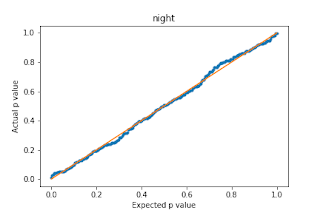## Posts

Showing posts from 2019

### L2 projection onto the probability simplex with bound constraints

Projecting onto the probability simplex is a common problem that arises in frequency estimation and related tasks.  This problem can be solved efficiently with an $O(n \log{(n)})$ algorithm.  In this blog post I consider the related problem of projecting onto the probability simplex with bound constraints.  Given a vector $\mathbf{r} \in \mathbb{R}^n$, our goal is to find a vector $\mathbf{p}^*$ that solves the following optimization problem. \begin{equation*} \begin{aligned} & \underset{\mathbf{p}}{\text{minimize}} & & \frac{1}{2} \lvert \lvert \mathbf{p} - \mathbf{r} \rvert \rvert_2^2 \\ & \text{subject to} & & \mathbf{1}^T \mathbf{p} = 1 \\ & & & \mathbf{a} \leq \mathbf{p} \leq \mathbf{b} \\ \end{aligned} \end{equation*} This problem generalizes the standard probability simplex projection problem by introducing arbitrary bound constraints $\mathbf{a} \leq \mathbf{p} \leq \mathbf{b}$. Here, $\mathbf{a}, \mathbf{b} \in \mathbb{R}^n$. I

### Beyond vectorization: writing efficient numpy code

Numpy is a great library for expressing computations over vectors, matrices, and multi-dimensional arrays in python due to it’s simplicity and efficiency. In a previous blog post , I discussed fairly well-known techniques for speeding up (and cleaning up) numpy code by avoiding loops and exploiting problem structure. I showed that when you use the suggestions in that post, you can speed up your numpy code by orders of magnitude. In this blog post, I’ll show you how to get even more speed out of your numpy code on top of the improvements you get from vectorization. As a simple example to illustrate an inefficiency of numpy, consider computations of the form z = 0.2*x + 0.8*y where x and y are large numpy arrays. This computation will form intermediate arrays 0.2*x and 0.8*y, so the memory overhead can be problematic and slow down the computation. On my laptop, it takes about 1 second for arrays of size 100 million: import numpy as np def foo(x,y): return 0.2*x + 0.

### Beat the Streak: Day SevenIn this blog post, I discuss questions of the form: “Does batter X perform better at home or away?   At day or night?   Against lefties or righties? On Friday or Monday?”   What I found was a little bit surprising.   Take for example the batter Daniel Murphy.   When you look at his data from 2011 - 2017, you will see that he got a hit in 29.85% of 1424 plate appearances during day games and he got a hit in 26.97% of 2673 plate appearances during night games.   This is a pretty meaningful difference, but is it statistically significant?   In other words, could this difference be explained purely by chance?   To answer this question, we can perform a chi squared test under the null hypothesis that the true probabilities are the same.   When we do this we get a chi squared value of 3.35 and a corresponding p value of 0.067.   Thus, we can reject the null hypothesis that the true underlying probabilities are the same at the 90% confidence level.   This is pretty convincing evidence th